LEVEL Statement

LEVEL <options> ;

The LEVEL statement is used to include a level component in the model. The level component, either by itself or together with a slope component (see the SLOPE statement), forms the trend component,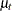, of the model. If the slope component is absent, the resulting trend is a random walk (RW) specified by the following equations: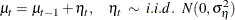If the slope component is present, signified by the presence of a SLOPE statement, a locally linear trend (LLT) is obtained. The equations of LLT are as follows: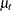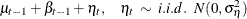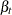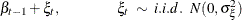In either case, the options in the LEVEL statement are used to specify the value of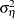and to request forecasts of. The SLOPE statement is used for similar purposes in the case of slope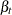. The following examples illustrate the use of the LEVEL statement. Assuming that a SLOPE statement is not added subsequently, a simple random walk trend is specified by the following statement:

      level;


The following statements specify a locally linear trend with value offixed at 4. It also requests printing of filtered values of. The value of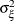, the disturbance variance in the slope equation, is estimated from the data.

      level variance=4 noest print=filter;
slope;

CHECKBREAK

turns on the checking of breaks in the level component.

NOEST

fixes the value ofto the value specified in the VARIANCE= option.

PLOT=FILTER
PLOT=SMOOTH
PLOT=( <FILTER> <SMOOTH> )

requests plotting of the filtered or smoothed estimate of the level component.

PRINT=FILTER
PRINT=SMOOTH
PRINT=( <FILTER> <SMOOTH> )

requests printing of the filtered or smoothed estimate of the level component.

VARIANCE=value

specifies an initial value for, the disturbance variance in theequation at the start of the parameter estimation process. Any nonnegative value, including zero, is an acceptable starting value.